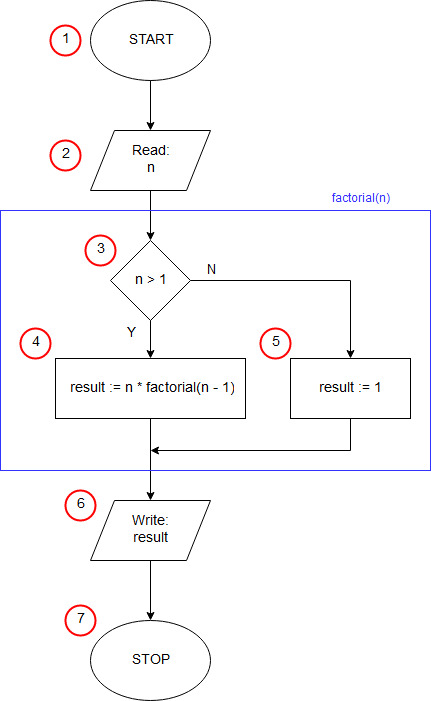# Factorial in C++ - recursive#include using namespace std; unsigned int factorial(unsigned int n) { if(n>1) return n*factorial(n-1); else return 1; } int main(int argc, char *argv[]) { unsigned int n, f; cout<<"n = "; cin>>n; f=factorial(n); cout<>c; }

In the first line of the program, we attach the iostream header file (input-output streams). This is necessary because in the further part of the program you will want to be able to write on the screen (cout) and load input data from the keyboard (cin). In the 2 line, we inform the compiler that we will use in the program names defined in the std namespace (they will be: cin, cout, endl), if we did not, it would be necessary to use longer qualified names (std::cin, std::cout, std::endl).

In line 4, we declare a function called 'factorial', taking one integer parameter (n) and returning the whole number as a result. Between the logical brackets { from the end of the line 4 and } on the line 9 there is a function body - a set of instructions executed by the program after calling this function.

In line 5, we check if n is greater than 1 - block (3) of the algorithm. If so, in line 6, we determine the result of the function as n multiplied by the value of the function of the engine (the same function as the one we currently define) for n minus 1 (4). At this point, the function factorial calls itself. This function is called a recursive function. If n is not greater than 1, then in line 8 we set the result of the function equal to 1 (ending condition of recursion) - block (5).

In C ++, the program starts with the main function. Line 11 contains the beginning of the definition of the main function, this is the beginning of the implementation of our algorithm (block START (1)). In the next line, we declare two integer variables (variables n and result).

The lines from13 to 14 perform the input/output operation (2), in which the input data is taken - the argument of the function 'factorial'.

In the line 15 the result of the function factorial() called with the argument n is assigned to variable f.

On line 16, we print the result obtained (input-output block (6)).

Instructions in lines 17 - 18 have no relation to the implementation of the algorithm. They are used to stop the program until you enter any character and press the enter key to prevent the program window from closing immediately.

The curly brace} from line 19 completes the main function and also the operation of the entire program (END block of the algorithm (7)).